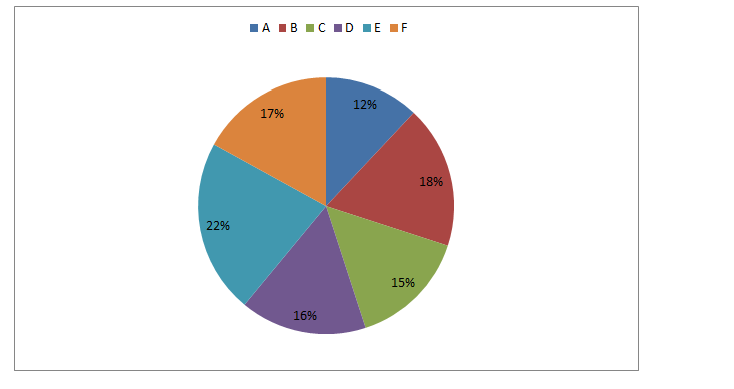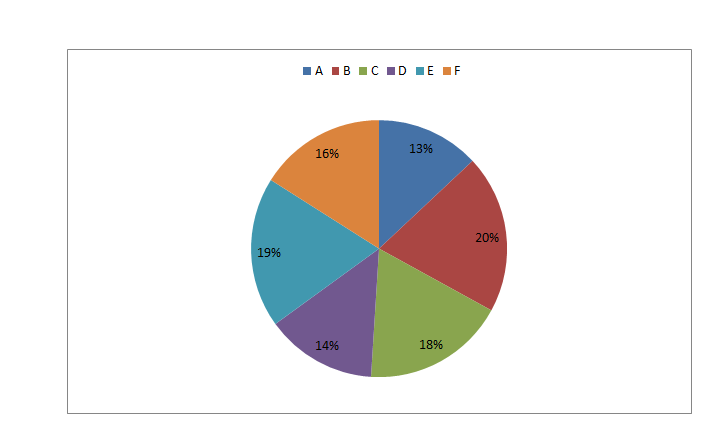# SBI PO Prelims Quantitative Aptitude Questions 2019 (Day-38)

Dear Aspirants, Our IBPS Guide team is providing new series of Quantitative Aptitude Questions for SBI PO 2019 so the aspirants can practice it on a daily basis. These questions are framed by our skilled experts after understanding your needs thoroughly. Aspirants can practice these new series questions daily to familiarize with the exact exam pattern and make your preparation effective.

[WpProQuiz 6214]

### Click Here for SBI PO Pre 2019 High-Quality Mocks Exactly on SBI Standard

Directions (Q. 1 – 5): What approximate value should come in place of (?) in the following questions?

1) 14 % of 2399 = 64 × 125.89 ÷ 7.21 – ? + 1900.25

a) 3278

b) 3560

c) 3944

d) 2716

e) 2952

2) (3.92/11) of 176.18 × 45.12 ÷ 14.78 – ? = 11.95

a) 180

b) 145

c) 120

d) 230

e) 255

3) (25.79)2 ÷ 13 × 7.21 + (24.89)2 = 4 × ?

a) 326

b) 192

c) 248

d) 384

e) 290

4) 27.5 % of 680 + 2.47 × 8.125 – ? = √3024.75

a) 286

b) 152

c) 244

d) 188

e) 320

5) (3/5) of 1564 + (26.12)2 = 30 % of ?

a) 4256

b) 4834

c) 6165

d) 5383

e) 5728

Directions (Q. 6 – 10): Study the following information carefully and answer the given questions.

The following pie chart1 shows the percentage distribution of total number of students enrolled for MBA from various institutions and the pie chart2 shows the percentage distribution of total number of students successfully completed the course.

Total students enrolled = 40000Total students successfully completed the course = 320006) Find the difference between the total number of students enrolled for MBA course from institute A and C together to that of total number of students successfully completed the course from institute B and D together?

a) 80

b) 150

c) 230

d) 320

e) None of these

7) Which institute has highest percentage of successful candidates out of the total number of enrolled candidates?

a) Institute D

b) Institute A

c) Institute B

d) Institute C

e) None of these

8) Find the ratio between the total number of candidates not completed the course from institute D to that of institute F?

a) 11 : 9

b) 17 : 13

c) 8 : 7

d) 9 : 5

e) None of these

9) Total number of candidates enrolled for MBA course from institute B and E together is approximately what percentage of total number of candidates successfully completed the course from institute A and C together?

a) 160 %

b) 145 %

c) 120 %

d) 105 %

e) 80 %

10) Find the difference between the average number of students enrolled for institute C, D and F together to that of the average number of students successfully completed the course from institute C, D and E together?

a) 1020

b) 840

c) 780

d) 960

e) None of these

(14/100) * 2400 = 64 * (126/7) – x + 1900

336 = 1152 – x + 1900

x = 1152 + 1900 – 336 = 2716

(4/11)*176*(45/15) – x = 12

192 – 12 = x

x = 180

(26*26*7)/13 + 252 = 4x

364 + 625 = 4x

4x = 989

X = 247.25 = 248

(55/200)*680 + (2.5*8) – x = √3025

187 + 20 – x = 55

X = 187 + 20 – 55 = 152

(3/5)*1565 + 262 = (30/100)*x

939 + 676 = (3x/10)

1615*(10/3) = x

X = 5383

The total number of students enrolled for MBA course from institute A and C together

= > 40000*(27/100) = 10800

The total number of students successfully completed the course from institute B and D together

= > 32000*(34/100) = 10880

Required difference = 10880 – 10800 = 80

Institute A = > Successful % = (4160/4800)*100 = 86.667 %

Institute B = > Successful % = (6400/7200)*100 = 88.889 %

Institute C = > Successful % = (5760/6000)*100 = 96 %

Institute D = > Successful % = (4480/6400)*100 = 70 %

Institute E = > Successful % = (6080/8800)*100 = 69.09 %

Institute F = > Successful % = (5120/6800)*100 = 75.29 %

Institute C has highest percentage of successful candidates.

The total number of candidates not completed the course from institute D

= > [40000*(16/100)] – [32000*(14/100)]

= > 6400 – 4480 = 1920

The total number of candidates not completed the course from institute F

= > [40000*(17/100)] – [32000*(16/100)]

= > 6800 – 5120 = 1680

Required ratio = 1920 : 1680 = 8 : 7

Total number of candidates enrolled for MBA course from institute B and E together

= > 40000*(40/100) = 16000

Total number of candidates successfully completed the course from institute A and C together

= > 32000*(31/100) = 9920

Required % = (16000/9920)*100 = 161.29 % = 160 %

The average number of students enrolled for institute C, D and F together

= > 40000*[(15 + 16 + 17)/100]*(1/3) = 6400

The average number of students successfully completed the course from institute C, D and E together

= > 32000*[(18 + 14 + 19)/100]*(1/3) = 5440

Required difference = 6400 – 5440 = 960